CAT  >  Maths and Logical Reasoning Test- 5

# Maths and Logical Reasoning Test- 5

Test Description

## 15 Questions MCQ Test TISS-NET Section Wise & Full Mock Test Series | Maths and Logical Reasoning Test- 5

Maths and Logical Reasoning Test- 5 for CAT 2022 is part of TISS-NET Section Wise & Full Mock Test Series preparation. The Maths and Logical Reasoning Test- 5 questions and answers have been prepared according to the CAT exam syllabus.The Maths and Logical Reasoning Test- 5 MCQs are made for CAT 2022 Exam. Find important definitions, questions, notes, meanings, examples, exercises, MCQs and online tests for Maths and Logical Reasoning Test- 5 below.
Solutions of Maths and Logical Reasoning Test- 5 questions in English are available as part of our TISS-NET Section Wise & Full Mock Test Series for CAT & Maths and Logical Reasoning Test- 5 solutions in Hindi for TISS-NET Section Wise & Full Mock Test Series course. Download more important topics, notes, lectures and mock test series for CAT Exam by signing up for free. Attempt Maths and Logical Reasoning Test- 5 | 15 questions in 15 minutes | Mock test for CAT preparation | Free important questions MCQ to study TISS-NET Section Wise & Full Mock Test Series for CAT Exam | Download free PDF with solutions
 1 Crore+ students have signed up on EduRev. Have you?
Maths and Logical Reasoning Test- 5 - Question 1

### A bag contains 5 green, 7 yellow, and 4 red balls. Three balls are drawn at random, find the probability that all the balls are of the same color.

Detailed Solution for Maths and Logical Reasoning Test- 5 - Question 1

Given: Green ball =5

Yellow ball =7

Red ball =4

Total ball =5+7+4=16

Probability that all the balls are of same colour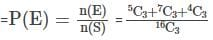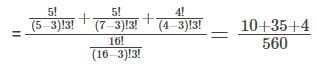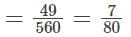Hence, the correct option is (D).

Maths and Logical Reasoning Test- 5 - Question 2

### The numerical value ofis ?

Detailed Solution for Maths and Logical Reasoning Test- 5 - Question 2

Given: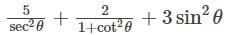We know that,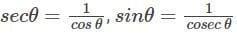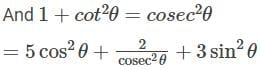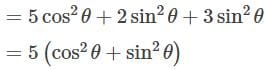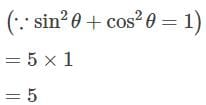Hence, the correct option is (A).

Maths and Logical Reasoning Test- 5 - Question 3

### P can do a piece of work in 15 days and Q in 20 days. With help of R, they finish the work in 5 days. In how many days R alone can do the same work?

Detailed Solution for Maths and Logical Reasoning Test- 5 - Question 3

P can do a piece of work in 15 days.

Part of work P can do in a day = 1/15

Q can do a piece of work in 20 days.

Part of work Q can do in a day = 1/20

Let, R can do a piece of work in x days.

Part of work R can do in a day = 1/x

P, Q and R together can do the work in 5 days.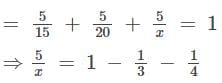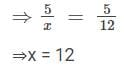∴ R can do a piece of work in 12 days.

Hence, the correct option is (B).

Maths and Logical Reasoning Test- 5 - Question 4

A cricketer scored some runs in his continuous 9 innings. He scored 100 runs in his 10th innings and increases his average by 8 runs. What was the average of his runs at the end of 10th innings?

Detailed Solution for Maths and Logical Reasoning Test- 5 - Question 4

Let, the average of an inning =x

Total runs scored in 9 innings =9x

Total runs scored in 10 innings =9x + 10

New average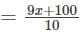since average increases by 8 ,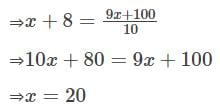Average after 10 innings =20+8=28

Hence, the correct option is (C).

Maths and Logical Reasoning Test- 5 - Question 5

A person buys several fruits at the rate of 16 for Rs 24 and sells them at the rate of 8 for Rs 18. What is his gain percent?

Detailed Solution for Maths and Logical Reasoning Test- 5 - Question 5

Given: Cost price of 16 fruits = Rs 24

Sale price of 8 fruits = Rs 18

∴ Sale price of 16 fruits = Rs 36

Profit = Sale price − Cost price

= 36 - 24 = Rs 12

Thus, required gain percent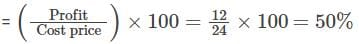Hence, the correct option is (A).

Maths and Logical Reasoning Test- 5 - Question 6

Find the simple interest on Rs. 3000 at 25/4% per annum for the period from 4th Feb, 2005 to 18th April, 2005.

Detailed Solution for Maths and Logical Reasoning Test- 5 - Question 6

Given:

Time = 4th Feb, 2005 to 18th April, 2005

= (24+ 31+18)days = 73 days

= 73/365 year = 1/5 year

P= Rs. 3000 and R = 25/4% p.a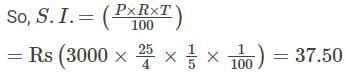Hence, the correct option is (D).

Maths and Logical Reasoning Test- 5 - Question 7

Direction: In following alphabet series, one term missing as shown by question mark. Choose missing term from options.

SGU, VJX, ?, BPD, ESG

Detailed Solution for Maths and Logical Reasoning Test- 5 - Question 7

This question follows the following pattern: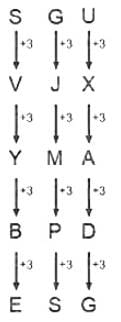So, YMA is the missing term in the series.

Hence, the correct option is (B).

Maths and Logical Reasoning Test- 5 - Question 8

Select the correct option that will fill in the blank and complete the series.

2, 3, 11, 38, __, 227, 443

Detailed Solution for Maths and Logical Reasoning Test- 5 - Question 8

Given series:

2, 3, 11, 38, __, 227, 443

The pattern followed is,

2 + 13 = 3

3 + 23 = 11

11 + 33 = 38

38 + 43 = 102

102 + 53 = 227

227 + 63 = 443

Hence, the correct option is (C).

Maths and Logical Reasoning Test- 5 - Question 9

Find the odd word from the given alternatives.

Detailed Solution for Maths and Logical Reasoning Test- 5 - Question 9

Each of the Teacher, Doctor and Lawyer can work independently but the Manager needs to be associated to a company whether his own or owned by someone else.

Hence, the correct option is (D).

Maths and Logical Reasoning Test- 5 - Question 10

The following equation is incorrect. Which two signs should be interchanged to correct the equation?

18 ÷ 2 - 25 × 9 + 6 = 38

Detailed Solution for Maths and Logical Reasoning Test- 5 - Question 10

Given:

18 ÷ 2 - 25 × 9 + 6 = 38

(A) + and × → 18 ÷ 2 – 25 + 9 × 6 = 38

(B) + and ÷ → 18 + 2 – 25 × 9 ÷ 6 = - 17.5

(C) - and + → 18 ÷ 2 + 25 × 9 – 6 = 228

(D) ÷ and × → 18 × 2 – 25 ÷ 9 + 6 = 39.2222

Hence, the correct option is (A).

Maths and Logical Reasoning Test- 5 - Question 11

Direction: What will come in place of the question mark (?) in the following number series?

17,18,57,290, ?

Detailed Solution for Maths and Logical Reasoning Test- 5 - Question 11

The series is:

17×1+1=18

18×3+3=57

57×5+5=290

290×7+7=2037

Hence, the correct option is (C).

Maths and Logical Reasoning Test- 5 - Question 12

A train of length 350 meters can cross a pole and platform of a certain length in 21 seconds and 36 seconds respectively, then what is the length of the platform?

Detailed Solution for Maths and Logical Reasoning Test- 5 - Question 12

Let the length of the platform and speed of train be x m and y km/h respectively.

According to the question: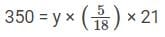y = 60 km/h

And,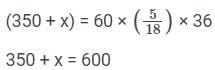x = 250 meters

Hence, the correct option is (B).

Maths and Logical Reasoning Test- 5 - Question 13

In the following question, find out the alternative which will replace the question mark.

Pediatricians : Children :: Podiatrists : ?

Detailed Solution for Maths and Logical Reasoning Test- 5 - Question 13

Pediatricians care for children from birth to young adulthood.

Similarly, Podiatrists care for problems in ankles and feet.

So, "ankle" is the correct answer.

Hence, the correct option is (A).

Maths and Logical Reasoning Test- 5 - Question 14

Find the minimum number of straight lines required to make the given figure.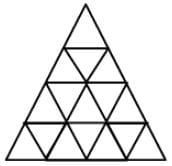Detailed Solution for Maths and Logical Reasoning Test- 5 - Question 14

We will label the figure as shown below: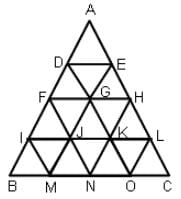The horizontal straight lines are BC,IL,FH and DE, i.e. 4.

The lines sloping to your left are IM,FN,DO and AC, i.e. 4.

The lines sloping to the right are LO,HN,EM and AB, i.e. 4.

Thus, the number of straight lines =3 x 4=12

Hence, the correct option is (B).

Maths and Logical Reasoning Test- 5 - Question 15

In the following question choose the appropriate option from the given alternatives.

Pigeon : Peace : : White flag : ?

Detailed Solution for Maths and Logical Reasoning Test- 5 - Question 15

Pigeon is a symbol of peace.

Similarly, the white flag is a symbol of surrender.

Hence, the correct option is (C).

## TISS-NET Section Wise & Full Mock Test Series

28 tests
 Use Code STAYHOME200 and get INR 200 additional OFF Use Coupon Code
Information about Maths and Logical Reasoning Test- 5 Page
In this test you can find the Exam questions for Maths and Logical Reasoning Test- 5 solved & explained in the simplest way possible. Besides giving Questions and answers for Maths and Logical Reasoning Test- 5, EduRev gives you an ample number of Online tests for practice

## TISS-NET Section Wise & Full Mock Test Series

28 tests

### How to Prepare for CAT

Read our guide to prepare for CAT which is created by Toppers & the best Teachers